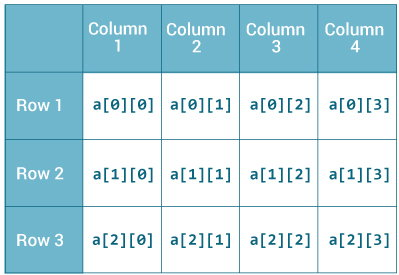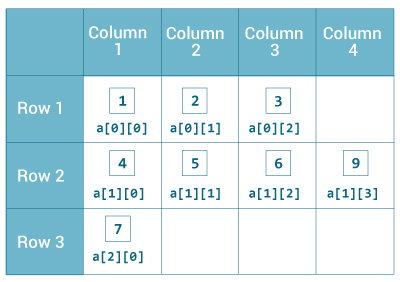# Java Multidimensional Arrays

In Java, you can declare an array of arrays known as multidimensional array.

Before learning multidimensional array, visit Java array article to learn about one-dimensional array.

In that chapter, you learned to create and use array of primitive data types (like: `Double`, `int` etc.), String array, and array of objects. It's also possible to create an array of arrays known as multidimensional array. For example,

`int[][] a = new int;`

Here, `a` is a two-dimensional (2d) array. The array can hold maximum of 12 elements of type `int`.Remember, Java uses zero-based indexing, that is, indexing of arrays in Java starts with 0 and not 1.

Similarly, you can declare a three-dimensional (3d) array. For example,

`String[][][] personalInfo = new String;`

Here, personalInfo is a 3d array that can hold maximum of 24 (3*4*2) elements of type `String`.

In Java, components of a multidimensional array are also arrays.

If you know C/C++, you may feel like, multidimensional arrays in Java and C/C++ works in similar way. Well, it doesn't. In Java, rows can vary in length.

You will see the difference during initialization.

## How to initialize a 2d array in Java?

Here's an example to initialize a 2d array in Java.

```int[][] a = {
{1, 2, 3},
{4, 5, 6, 9},
{7},
};
```

As mentioned, each component of array a is an array in itself, and length of each rows is also different.Let's write a program to prove it.

``````class MultidimensionalArray {
public static void main(String[] args) {

int[][] a = {
{1, 2, 3},
{4, 5, 6, 9},
{7},
};

System.out.println("Length of row 1: " + a.length);
System.out.println("Length of row 2: " + a.length);
System.out.println("Length of row 3: " + a.length);
}
}``````

When you run the program, the output will be:

```Length of row 1: 3
Length of row 2: 4
Length of row 3: 1```

Since each component of a multidimensional array is also an array (`a`, `a` and `a` are also arrays), you can use `length` attribute to find the length of each rows.

### Example: Print all elements of 2d array Using Loop

``````class MultidimensionalArray {
public static void main(String[] args) {

int[][] a = {
{1, -2, 3},
{-4, -5, 6, 9},
{7},
};

for (int i = 0; i < a.length; ++i) {
for(int j = 0; j < a[i].length; ++j) {
System.out.println(a[i][j]);
}
}
}
}``````

It's better to use for..each loop to iterate through arrays whenever possible. You can perform the same task using `for..each` loop as:

``````class MultidimensionalArray {
public static void main(String[] args) {

int[][] a = {
{1, -2, 3},
{-4, -5, 6, 9},
{7},
};

for (int[] innerArray: a) {
for(int data: innerArray) {
System.out.println(data);
}
}
}
}
``````

When you run the program, the output will be:

```1
-2
3
-4
-5
6
9
7
```

## How to initialize a 3d array in Java?

You can initialize 3d array in similar way like a 2d array. Here's an example:

```// test is a 3d array
int[][][] test = {
{
{1, -2, 3},
{2, 3, 4}
},
{
{-4, -5, 6, 9},
{1},
{2, 3}
}
};```

Basically, 3d array is an array of 2d arrays.

Similar like 2d arrays, rows of 3d arrays can vary in length.

### Example: Program to print elements of 3d array using loop

``````class ThreeArray {
public static void main(String[] args) {

// test is a 3d array
int[][][] test = {
{
{1, -2, 3},
{2, 3, 4}
},
{
{-4, -5, 6, 9},
{1},
{2, 3}
}
};

// for..each loop to iterate through elements of 3d array
for (int[][] array2D: test) {
for (int[] array1D: array2D) {
for(int item: array1D) {
System.out.println(item);
}
}
}
}
}
``````

When you run the program, the output will be:

```1
-2
3
2
3
4
-4
-5
6
9
1
2
3```